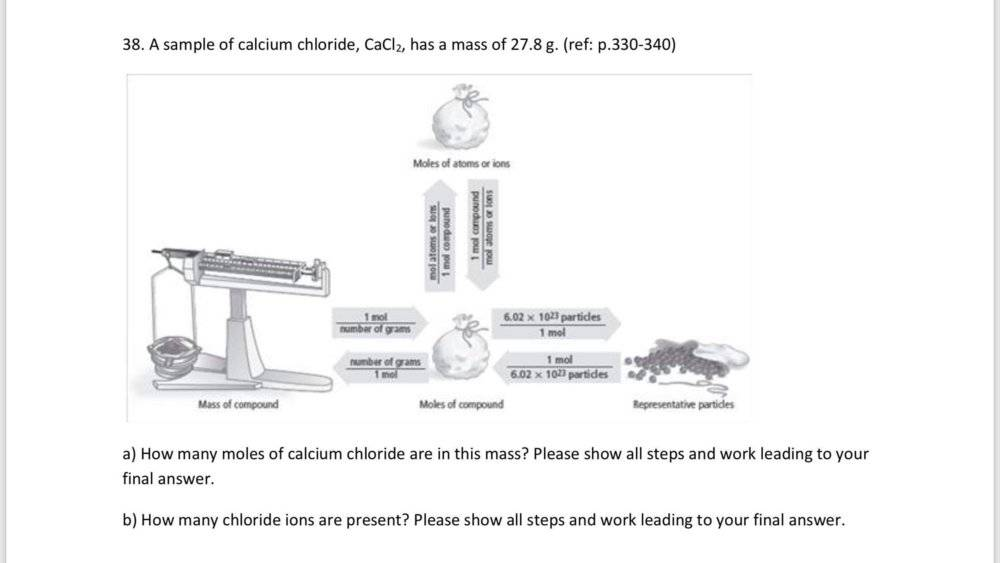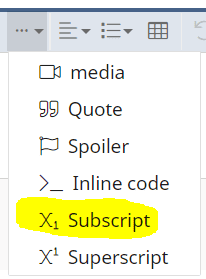# Calculating Number of Moles & Ions in CaCI2

• Chemistry
In summary, this student has difficulty with basic arithmetic and is struggling with some of the more tedious exercises in the book.f

Homework Statement
I have a question
Relevant Equations
N/A
Here is my work** Can someone please tell me if this is correct?

mass = 27.8 g CaCI2
Number of CaCI2 in the compound = (unknown)
Number of CI- ions in the compound = (unknown)
Number of moles = mass/molar mass
To find the number of moles of CaCI2 first you find the molar mass of the compound.
1 mole Ca x 40.08 g Ca/1 mole Ca = 40.08 g
1 mole CI x 30.45 g CI/1 mole CI = 30.45 g
1 mole CI x 30.45 g CI/1 mole CI = 30.45 g
molar mass = (40.08 g + 35.45 g + 35.45 g) = 110.98 g/mol CaCI2
27.8 g CaCI2 x 1 mol CaCI2/110.98 g CaCI2 = 0.250 mol CaCI2
Now from equation No of Chloride ions are : 6.02 x 10^23 x 2 CI- ions =
1.204 x 10^23 CI- ions•dshoup
Homework Statement:: I have a question you mean: 'see below for the problem statement'
Homework Equations:: N/A Oh ?

Now from equation No of Chloride ions are : 6.02 x 10^23 x 2 CI- ions =
1.204 x 10^23 CI- ions
can you explain this ?

PS kudos for dealing with the dimensions properly in part a) !

Homework Statement:: I have a question
Homework Equations:: N/A

mass = 27.8 g CaCI2
Number of CaCI2 in the compound = (unknown)
Number of CI- ions in the compound = (unknown)
Please be more careful in writing the chemical symbols, particularly when you write I in place of l (lower-case letter ell).
##CI_2## would be a compound made up of a carbon (C) atom and two atoms of Iodine (I), which might or might not be an actual compound. Chlorine's symbol is Cl, not CI.

In addition there is a subscript entry in the ... drop down menu :CaCl2 is so much easier on the eyeYou have calculated the number of Cl- ions in a mole of CaCl2.
The job was to calculate the number in what is approximately ¼ mole of it.
Is it that difficult?Is it difficult to see the answer is wrong?

It seems to me in several exercises you are making heavy weather of some rather tedious and pedantic exercises. All that is involved is some simple proportions. I suggest if necessary you revise on an elementary arithmetic book. (OK you need the concept of moles, Dalton's number, chemical compound.) But maybe you are making such a bad mistakes because it is very boring and mind-numbing. Maybe the way out is to tell yourself I want to learn about and understand some real chemistry. E.g. About these elements and compounds you are doing these calculations on. And read ahead in the textbook (hoping it is not all like this)!

•BvU